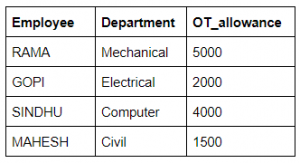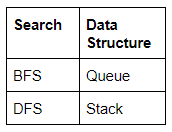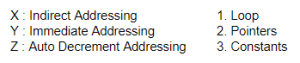## ISRO-2017 May

 Question 1
If A is a skew-symmetric matrix, then AT
 A diagonal matrix B A C -A D 0
Engineering-Mathematics       Linear-Algebra       Video-Explanation
Question 1 Explanation:
→ In mathematics, particularly in linear algebra, a skew-symmetric (or antisymmetric or antimetric) matrix is a square matrix whose transpose equals its negative, that is, it satisfies the condition.
→ If A is skew symmetric matrix then AT = -A
→ In terms of the entries of the matrix, if aij denotes the entry in the ith row and jth column, then the skew-symmetric condition is equivalent to
→ If A is skew symmetric matrix then aji=-aij
 Question 2
If A and B be two arbitrary events, then
 A P(A∩B) = P(A)P(B) B P(A∪B) = P(A) + P(B) C P(A|B) = P(A ∩ B) + P(B) D P(A∪B) <= P(A) + P(B)
Engineering-Mathematics       Probability       Video-Explanation
Question 2 Explanation:
(A) Happens when A and B are independent.
(B) Happens when A and B are mutually exclusive.
(C) Not happens.
(D) P(A∪B) ≤ P(A) + P(B) is true because P(A∪B) = P(A) + P(B) - P(A∩B).
 Question 3
Using Newton Raphson method, a root correct to 3 decimal places of the equation x3 – 3x – 5 = 0
 A 2.222 B 2.275 C 2.279 D None of the above
Engineering-Mathematics       Newton-Raphson-Method       Video-Explanation
Question 3 Explanation:
Here f(x)= x3-3x-5 and f1(x) = 3x2-3
The Newton-Raphson iterative formula isSince x3 and x4 are identical upto 3 places of decima,we take x4=2.279 as the required root, correct to three places of the decimal.
 Question 4
What does the data dictionary identify?
 A Field names B Field Formats C Field Types D All of these
Database-Management-System       Relational-databases       Video-Explanation
Question 4 Explanation:
→ A data dictionary(or) metadata repository, as defined in the IBM Dictionary of Computing, is a "centralized repository of information about data such as meaning, relationships to other data, origin, usage, and format".
→ Oracle defines it as a collection of tables with metadata. The term can have one of several closely related meanings pertaining to databases and database management systems (DBMS)
1. A document describing a database or collection of databases
2. An integral component of a DBMS that is required to determine its structure
3. A piece of middleware that extends or supplants the native data dictionary of a DBMS
 Question 5
Which of the following concurrency control protocol ensures both conflict serializability and free from deadlock?
 A Timestamp ordering B 2 Phase Locking C Both (a) and (b) D None of the above
Database-Management-System       Transactions       Video-Explanation
Question 5 Explanation:
→ Two-phase locking protocol (2PL) ensures the conflict serializable schedule but it may not free from deadlock.
→ Timestamp ordering protocol ensures conflict serializability and free from deadlock.
 Question 6
ACID properties of a transactions are
 A Atomicity, consistency, isolation, database B Atomicity, consistency, isolation, durability C Atomicity, consistency, integrity, durability D Atomicity, consistency, integrity, database
Database-Management-System       Transactions       Video-Explanation
Question 6 Explanation:
Atomicity: Execute the all the operations or none of them
→ Responsible for Transaction Manager
Consistency: Database should be consistent before and after the execution of the transaction
→ Responsible for user/application manager
Isolation: Each transaction Tie must be executed without knowing what is happening with other transactions. Responsible for Concurrency control manager
Durability: All updates done by a transaction must become permanent.
→ Responsible for recovery manager
 Question 7
Database table by name overtime_allowance is given belowWhat is the output of the following SQL query?
select count(*) from ((select Employee, Department from Overtime_allowance) as S
natural join (select Department, OT_allowance from Overtime_allowance) as T);
 A 16 B 4 C 8 D None of the above
Database-Management-System       SQL       Video-Explanation
Question 7 Explanation:Common attributes in both the table column are the department. So, we apply natural join, it will give the output as common tuples in both the table S and R.
 Question 8
Which symbol denote derived attributes in the ER Model?
 A Double ellipse B Dashed ellipse C Squared ellipse D An ellipse with attribute name underlined
Database-Management-System       ER-Model       Video-Explanation
Question 8 Explanation:
Derived attributes are depicted by dashed ellipse.
Example:Question 9
The symmetric difference of sets A = {1, 2, 3, 4, 5, 6, 7, 8} and B = {1, 3, 5, 6, 7, 8, 9} is
 A {1, 3, 5, 6, 7, 8} B {2, 4, 9} C {2, 4} D {1, 2, 3, 4, 5, 6, 7, 8, 9}
Engineering-Mathematics       Sets-And Relation       Video-Explanation
Question 9 Explanation:
Symmetrical Difference of A and B=(A–B)∪(B–A)Question 10
The problems 3-SAT and 2-SAT are
 A Both NP-complete B Both in P C NP-complete and in P, respectively D Undecidable and NP-complete, respectively
Algorithms       NP complete       Video-Explanation
Question 10 Explanation:
→ 2-SAT is the first non polynomial problem is solved in polynomial time.
→ 3-SAT problem is NP- complete problem
 Question 11
Given the following statements:
S1 : Every context-sensitive language L is recursive
S2 : There exists a recursive language that is not context-sensitive
Which statements are true?
 A Only S1 is correct B Only S2 is correct C Both S1 and S2 are not correct D Both S1 and S2 are correct
Theory-of-Computation       Languages-and-Grammars       Video-Explanation
Question 11 Explanation:
According to the Chomsky hierarchy, both the statements are correct.Question 12
Which one of the following is FALSE?
 A There is a unique minimal DFA for every regular language B Every NFA can be converted to an equivalent PDA C The complement of every context-free language is recursive D Every non-deterministic PDA can be converted to an equivalent deterministic PDA
Theory-of-Computation       Equivalence-of-Languages       Video-Explanation
Question 12 Explanation:
→ NPDA is more powerful than DPDA because
1. DPDA accept only a proper subset of CFL's ie. LL grammars.
2. NPDA can accept any CFL, which makes them more powerful over DPDA
3. Every NPDA can not be converted to an equivalent DPDA
 Question 13
In some programming languages, an identifier is permitted to be a letter followed by any number of letters or digits. If L and D denotes the set of letters and digit respectively. Which of the following expression defines an identifier?
 A (L + D)* B (L.D)* C L(L + D)* D L(L.D)*
Theory-of-Computation       Regular-Expression       Video-Explanation
Question 13 Explanation:
Option C is correct because it indicates L followed by (L + D)* where * means 0 or more occurences of L or D.
 Question 14
The recurrence relation that arises in relation with the complexity of binary search is:
 A T(n)=2T(n/2)+k , where k is constant B T(n)=T(n/2) +k, where k is constant C T(n)=T(n/2)+logn D T(n)=T(n/2)+n
Algorithms       Searching       Video-Explanation
Question 14 Explanation:
Binary search in a sorted array
The time to search in an array of ‘n’ elements is equal to the time to search in an array of n/2 elements plus k comparison.
T(n)=T(n/2)+k // k is constant
 Question 15
Which one of the following in-place sorting algorithms needs the minimum number of swaps?
 A Insertion Sort B Quick Sort C Heap Sort D Selection Sort
Algorithms       Sorting       Video-Explanation
Question 15 Explanation:
Selection sort requires minimum number of swaps i.e O(n)Question 16
Given two statements:
(i) Insertion of an element should be done at the last node in a circular list
(ii) Deletion of an element should be done at the last node of the circular list
 A Both are true B Both are false C First is false and second is true D None of the above
Question 16 Explanation:
There are three situation for inserting element and deleting an element in Circular linked list.
1.Insertion at the front of Circular linked list.
2.Insertion in the middle of the Circular linked list.
3.Insertion at the end of the Circular linked list.
 Question 17
Which of the following data structure is useful in traversing a given graph by breadth-first search?
 A Stack B List C Queue D None of the above
Data-Structures       Graphs       Video-Explanation
Question 17 Explanation:Question 18
How many 128×8 bit RAMs are required to design 32K×32 bit RAM?
 A 512 B 1024 C 128 D 32
Digital-Logic-Design       RAM       Video-Explanation
Question 18 Explanation:
Step-1: RAM size required is 32Kx32 bits
= 32x1024x32 bits. [Note: 1k=1024 bytes]

Step-2: Given RAM chip capacity is 128x8 bits
Step-3: Required size/Given size
=(32*1024*32) / (128*8)
=1024
 Question 19
The most appropriate matching for the following pairs :A X − 3, Y − 2, Z −1 B X − 2, Y − 3, Z −1 C X − 3, Y −1, Z − 2 D X − 2, Y −1, Z − 3
Question 19 Explanation:
Indirect addressing means that the address of the data is held in an intermediate location so that the address is first 'looked up' and then used to locate the data itself.
Immediate Addressing. An immediate operand has a constant value or an expression. When an instruction with two operands uses immediate addressing, the first operand may be a register or memory location, and the second operand is an immediate constant. Auto increment or decrements: can be one by using loops.
 Question 20
Which interrupt in 8085 Microprocessor is unmaskable?
 A RST 5.5 B RST 7.5 C TRAP D Both (a) and (b)
Computer-Organization       Microprocessor       Video-Explanation
Question 20 Explanation:
Interrupts are the signals generated by the external devices to request the microprocessor to perform a ask. There are 5 interrupt signals. Given low priority to high is
1. INTR
2. RST 5.5
3. RST 6.5
4. RST 7.5
5. TRAP
Maskable Interrupts: They can be enabled or disabled by software INTR,RST 5.5,RST 6.5 and RST 7.5